NCERT Solution (Part - 3) - Accounting Ratios

# NCERT Solution (Part - 3) - Accounting Ratios - Notes | Study Additional Study Material for Commerce - Commerce

 1 Crore+ students have signed up on EduRev. Have you?
Page No. : 241
Numerical Questions :
Question 1 : Calculate Inventory Turnover Ratio from the data given below: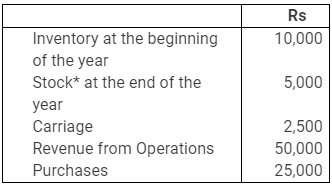*Since the very first item is Inventory in the beginning, so this item should be Inventory at the end.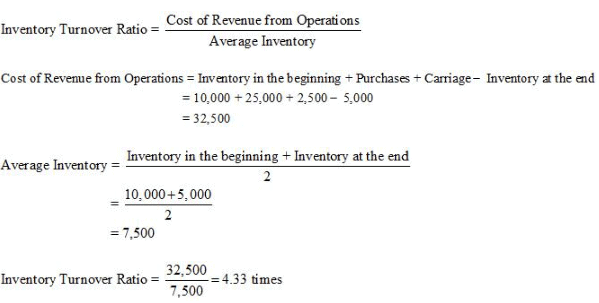Question 2 : A trading firm’s average inventory is Rs 20,000 (cost). If the inventory turnover ratio is 8 times and the firm sells goods at a profit of 20% on sale, ascertain the profit of the firm.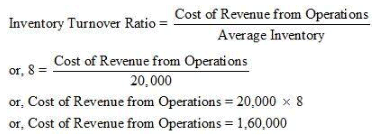Let Sale Price be Rs 100
Then Profit is Rs 20
Hence, the Cost of Revenue from Operations = Rs 100 − Rs 20 = Rs 80
If the Cost of Revenue from Operations is Rs 80, then Revenue from Operations = 100
If the Cost of Revenue from Operations is Rs 1, then Revenue from Operations = 100/80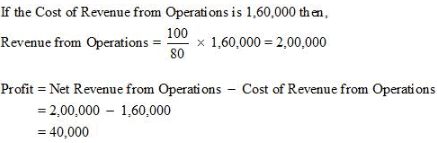Question 3 : You are able to collect the following information about a company for two years: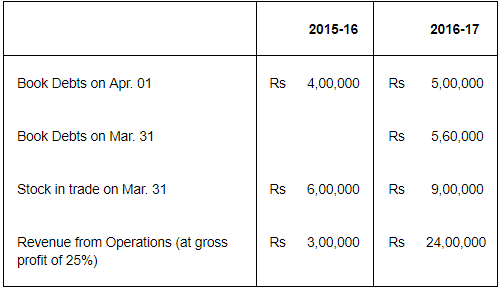Calculate Inventory Turnover Ratio and Trade Receivables Turnover Ratio if in the year 2015-16 stock in trade increased by Rs 2,00,000.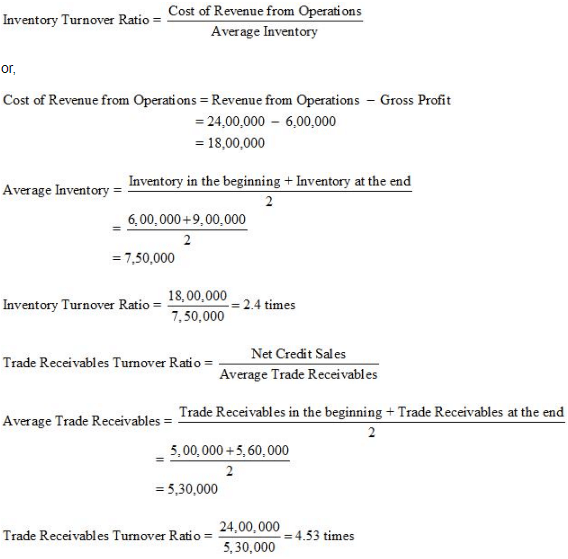Note: It has been assumed that all sales are credit sales
Page no. 242
Numericals Questions
Question 1 : The following Balance Sheet and other information, calculate following ratios:
(i) Debt-Equity Ratio
(ii) Working Capital Turnover Ratio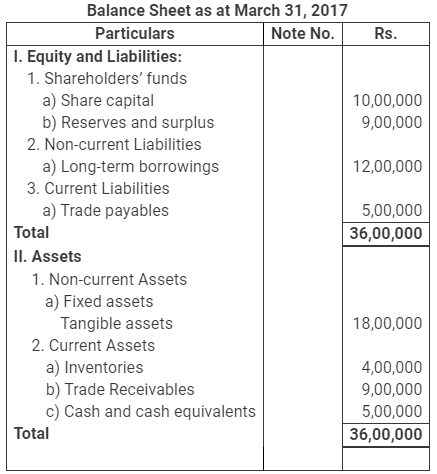Additional Information: Revenue from Operations Rs. 18,00,000 Calculate:
i) Debt-Equity Ratio
ii) Working Capital Turnover Ratio
(Debt-Equity Ratio 0.63:1; Working Capital Turnover Ratio 1.39 times; Trade
Receivables Turnover Ratio 2 times)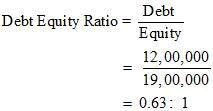Debt = Long Term Borrowings = Rs 12,00,0000
Equity = Share Capital + Reserve and Surplus
= 10,00,000 + 9,00,000
= Rs 19,00,000
2. Working Capital Turnover Ratio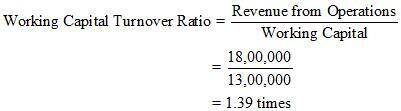Revenue from Operations = Rs 18, 00,000
Working Capital = Current Assets – Current Liabilities
= 18,00,000 – 5,00,000
= Rs 13,00,000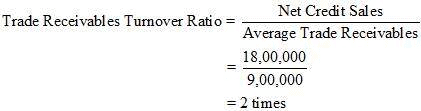Net Credit Sales = Rs 18,00,000
Average Trade Receivables = Rs 9,00,000
Notes:
1. Revenue from Operations are assumed to be revenue generated from credit sales.

2. The amount of trade receivables given in the Balance Sheet is assumed to be Average Trade Receivables.

Question 2 : From the following information, calculate the following ratios:
i) Quick Ratio
ii) Inventory Turnover Ratio
iii) Return on Investment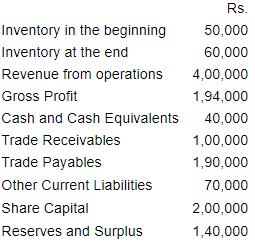(Balance in the Statement of Profit & Loss A/c)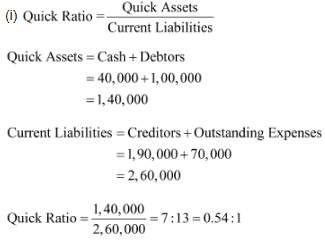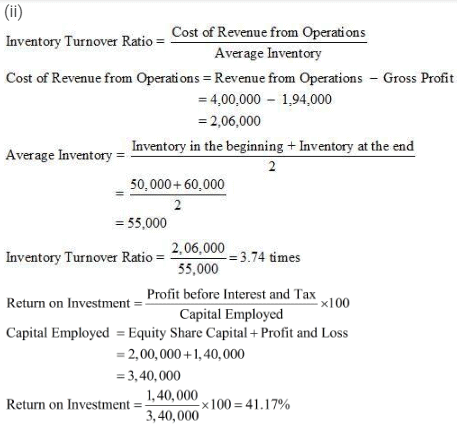Page no. 243
Numerical Questions :
Question 3 : From the following, calculate
(a) Debt Equity Ratio (b) Total Assets to Debt Ratio (c) Proprietary Ratio.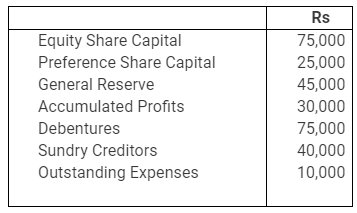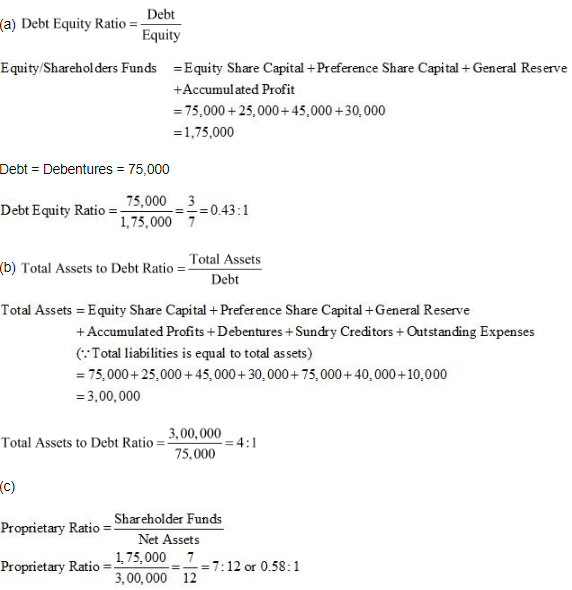Question 4 : Cost of Revenue from Operations is Rs 1,50,000. Operating expenses are Rs 60,000. Revenue from Operations is Rs 2,50,000. Calculate Operating Ratio.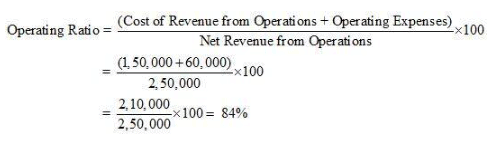Question 5 : The following is the summerised transactions and Statement of Profit and Loss Account for the year ending March 31, 2007 and the Balance Sheet as on the basis of following information, calculate:
(i) Gross Profit Ratio (ii) Current Ratio (iii) Acid Test Ratio (iv) Inventory Turnover Ratio (v) Fixed Assets Turnover Ratio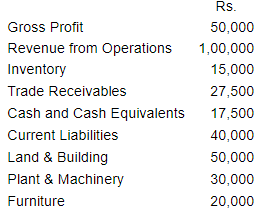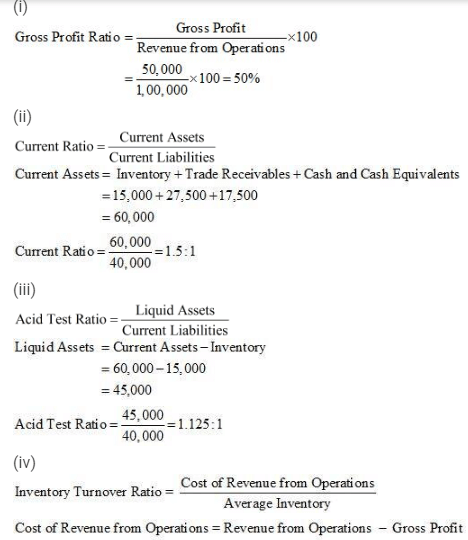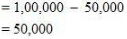Average Inventory = 15,000*
*Note: Since values for inventory in the beginning and inventory at the end is not given, the amount of inventory is assumed to be average inventory.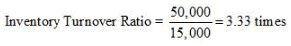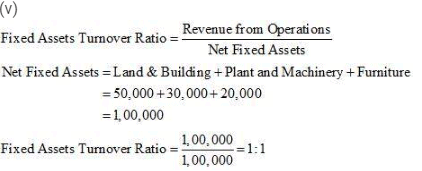Question 6 : From the following information calculate Gross Profit Ratio, Inventory Turnover Ratio and Trade Receivables Turnover Ratio.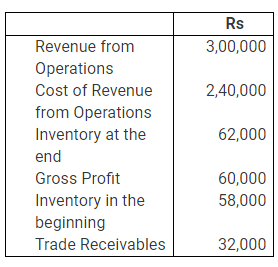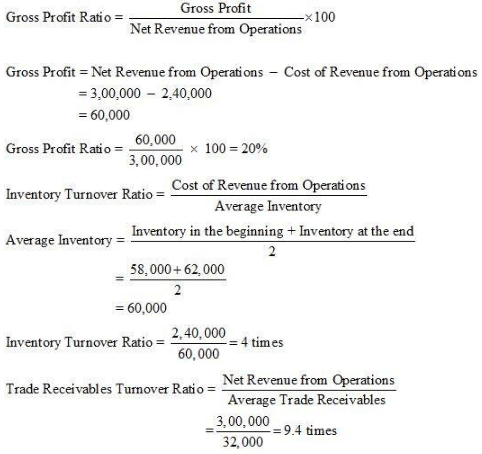Note: In the solution, Trade Receivables are assumed as the Average Trade Receivables.

The document NCERT Solution (Part - 3) - Accounting Ratios - Notes | Study Additional Study Material for Commerce - Commerce is a part of the Commerce Course Additional Study Material for Commerce.
All you need of Commerce at this link: Commerce

## Additional Study Material for Commerce

4 videos|168 docs

## Additional Study Material for Commerce

4 videos|168 docs

Track your progress, build streaks, highlight & save important lessons and more!

,

,

,

,

,

,

,

,

,

,

,

,

,

,

,

,

,

,

,

,

,

;